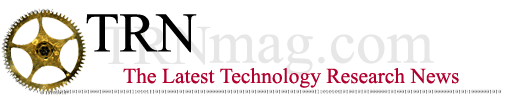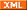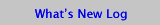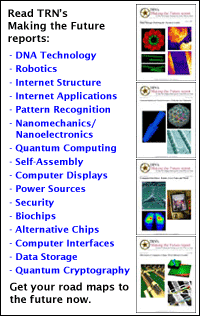## Quantum data compares faster

By Kimberly Patch, Technology Research News

Although computers that use quantum particles like atoms or electrons to represent the ones and zeros of computing are at least two decades from practical reality, researchers are finding that quantum computers can theoretically compute exponentially faster than the fastest possible electronic computer -- at least for some tasks.

Researchers from Canada and the Netherlands have found a mathematical fingerprinting scheme that would allow quantum computers to compare two sets of data much more efficiently than is possible with the classical computers we use today.

This quantum fingerprinting scheme increases the list of mathematical problems that quantum computers would be able to solve much faster than classical computers. "It gives an example of a fairly natural problem where quantum communication is exponentially more efficient than classical communication," said Ronald de Wolf, who was a researcher at the Center for Mathematics and Computer Science in the Netherlands when the work was done, but is now at the University of California at Berkeley. Other examples include factoring large numbers to crack secret codes and searching large databases.

The fingerprinting scheme could eventually be used to produce efficient communications among quantum computers, and also in quantum cryptography, said de Wolf.

Quantum computers use different states of quantum particles like atoms or electrons to represent the ones and zeros of computing as quantum bits, or qubits. For example, an electron can be spin up, or spin down in a way similar to a top which spins either clockwise or counterclockwise.

The fingerprinting scheme essentially allows researchers to make a much smaller mathematical fingerprint of a set of data. It takes less computing power and communications bandwidth to work with the fingerprints rather than the full data sets to, for instance, compare them. The quantum scheme allows a mathematical fingerprint of a set of data to be more than an order of magnitude smaller than would be possible using today's classical computers.

The mathematics involves three steps. First, the data is plotted in an imaginary, many-dimensional space.

Second, the information in the many-dimensional space is boiled down, or fingerprinted, using only a small number of qubits. The number of qubits needed is equal to the logarithm of the number of dimensions involved. The logarithm of a number, which is the number of times 10 must be multiplied together to equal that number, increases very slowly relative to the size of the number. For example, the logarithm of 10 is 1 and the logarithm of 100 is 2.

Third, it is possible to test whether two given quantum states are equal, which allows two data sets plotted and fingerprinted this way to be compared. "There is no classical analog to the second or third [steps]," making it impossible for classical computers to do this,just delete" said de Wolf.

Instead, today's computers require a number of bits equal to the square root of the number of dimensions, which is a much larger number than the logarithm. For example, the square root of 10 is 3.16 and the square root of 100 is 10.

For a 1-megabyte set of data, a classical computer fingerprint would require 3,000 bits, while the quantum fingerprint would be only 25 quantum bits long. The gap widens as the data set grows. For a 1,000-gigabyte set of data, a classical fingerprint would take up 3 million bits, and the quantum scheme only 45 quantum bits. There are eight bits in one byte of data.

What makes qubits mathematically more flexible has to do with the weird quantum properties of particles. Rather than simply representing a 1 or a 0, a qubit is a superposition of both, meaning it has a certain probability of being a 0 and a certain probability of being a 1. The two possibilities are complex numbers whose squared values add up to the total of both. "The point of quantum bits... is that their state can be partly zero and partly one at the same time. Mathematically... it's this superposition of things that would exclude each other in the classical world that matters," said de Wolf.

What the mathematics boils down to is the quantum fingerprinting scheme exponentially reduces the amount of communication required for comparing sets of data, said de Wolf.

For example, if Alice and Bob were on distance spaceships that could not communicate with each other, but could only send messages to a command center on earth, in order to compare two large sets of data from Alice and Bob, the command center would only require Alice and Bob to send the fingerprints rather than the full data set, de Wolf said.

It's exciting work, said Emanuel Knill, a mathematician at Los Alamos National Laboratory. It is "one way in which two people with large documents but limited communication can efficiently determine whether the documents or the same are not." The work establishes that quantum computing could be exponentially more efficient in this type of communications than today's computers, he added.

The researchers could implement the work in the laboratory within a few years, according to de Wolf. The quantum computers that would use the scheme, however, are further off. Most researchers agree it will be at least 20 years before practical quantum computers could be built.

De Wolf's research colleagues were Harry Berman of the University of Amsterdam and the Center for Mathematics and Computer Science (CWI) in the Netherlands, and Richard Cleve and John Watrous of the University of Calgary in Canada. They published the work in Physical Review Letters, September 26, 2001. The research was funded by the European Union and Natural Sciences and Engineering Research Council of Canada (NSERC).

Timeline:   3 years, > 20 years
Funding:   Government
TRN Categories:   Quantum Computing
Story Type:   News
Related Elements:  Technical paper, "Quantum Fingerprinting," Physical Review Letters, September 2001, also posted in the arXiv physics archive at http://arXiv.org/abs/quant-ph/0102001.

January 23, 2002

Page One

Laser speeds data through air

Nanotube array could form chips

Hot spots give away lying eyes

Quantum data compares faster

Artificial crystals change laser colors

News:

Research News Roundup
Research Watch blog

Features:
View from the High Ground Q&A
How It Works

News| Blog| BooksAdvertisements: